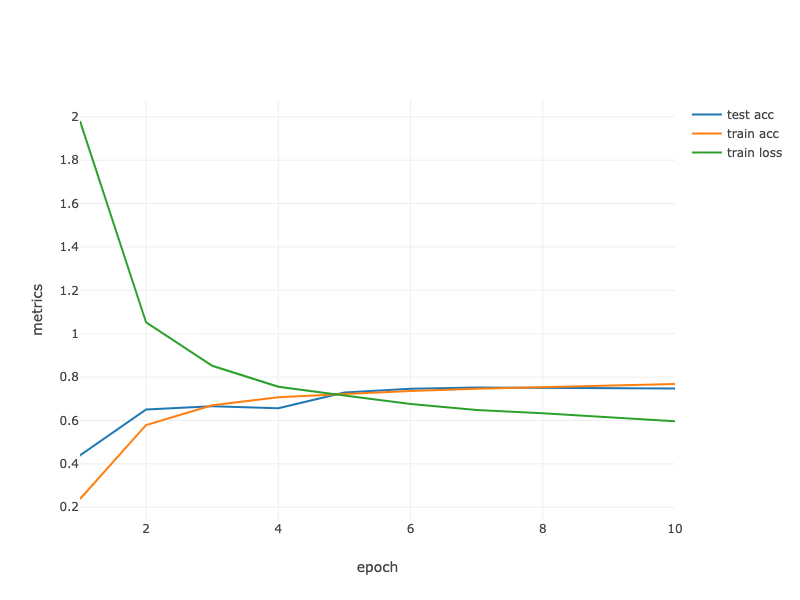# 6.6. 卷积神经网络（LeNet）¶

## 6.6.1. LeNet¶Fig. 6.6.1 Data flow in LeNet 5. The input is a handwritten digit, the output a probability over 10 possible outcomes.

%load ../utils/djl-imports

import ai.djl.basicdataset.cv.classification.*;
import ai.djl.metric.*;
import org.apache.commons.lang3.ArrayUtils;

Engine.getInstance().setRandomSeed(1111);

NDManager manager = NDManager.newBaseManager();

SequentialBlock block = new SequentialBlock();

block
.setKernelShape(new Shape(5, 5))
.optBias(false)
.setFilters(6)
.build())
.add(Pool.avgPool2dBlock(new Shape(5, 5), new Shape(2, 2), new Shape(2, 2)))
.setKernelShape(new Shape(5, 5))
.setFilters(16).build())
.add(Pool.avgPool2dBlock(new Shape(5, 5), new Shape(2, 2), new Shape(2, 2)))
// Blocks.batchFlattenBlock() 将转换形状的输入（批次大小、通道、高度、宽度）
// 输入形状（批量大小,通道*高度*宽度）
.builder()
.setUnits(120)
.build())
.builder()
.setUnits(84)
.build())
.builder()
.setUnits(10)
.build());

SequentialBlock {
Conv2d
LambdaBlock
avgPool2d
Conv2d
LambdaBlock
avgPool2d
batchFlatten
Linear
LambdaBlock
Linear
LambdaBlock
Linear
}


float lr = 0.9f;
Model model = Model.newInstance("cnn");
model.setBlock(block);

Loss loss = Loss.softmaxCrossEntropyLoss();

Tracker lrt = Tracker.fixed(lr);
Optimizer sgd = Optimizer.sgd().setLearningRateTracker(lrt).build();

DefaultTrainingConfig config = new DefaultTrainingConfig(loss).optOptimizer(sgd) // 优化器（损失函数）
.optDevices(Engine.getInstance().getDevices(1)) // 单个GPU

Trainer trainer = model.newTrainer(config);

NDArray X = manager.randomUniform(0f, 1.0f, new Shape(1, 1, 28, 28));
trainer.initialize(X.getShape());

Shape currentShape = X.getShape();

for (int i = 0; i < block.getChildren().size(); i++) {
Shape[] newShape = block.getChildren().get(i).getValue().getOutputShapes(new Shape[]{currentShape});
currentShape = newShape;
System.out.println(block.getChildren().get(i).getKey() + " layer output : " + currentShape);
}

01Conv2d layer output : (1, 6, 28, 28)
02LambdaBlock layer output : (1, 6, 28, 28)
03LambdaBlock layer output : (1, 6, 14, 14)
04Conv2d layer output : (1, 16, 10, 10)
05LambdaBlock layer output : (1, 16, 10, 10)
06LambdaBlock layer output : (1, 16, 5, 5)
07LambdaBlock layer output : (1, 400)
08Linear layer output : (1, 120)
09LambdaBlock layer output : (1, 120)
10Linear layer output : (1, 84)
11LambdaBlock layer output : (1, 84)
12Linear layer output : (1, 10)Fig. 6.6.2 Compressed notation for LeNet5

## 6.6.2. 数据采集和训练¶

int batchSize = 256;
int numEpochs = Integer.getInteger("MAX_EPOCH", 10);
double[] trainLoss;
double[] testAccuracy;
double[] epochCount;
double[] trainAccuracy;

epochCount = new double[numEpochs];

for (int i = 0; i < epochCount.length; i++) {
epochCount[i] = (i + 1);
}

FashionMnist trainIter = FashionMnist.builder()
.optUsage(Dataset.Usage.TRAIN)
.setSampling(batchSize, true)
.optLimit(Long.getLong("DATASET_LIMIT", Long.MAX_VALUE))
.build();

FashionMnist testIter = FashionMnist.builder()
.optUsage(Dataset.Usage.TEST)
.setSampling(batchSize, true)
.optLimit(Long.getLong("DATASET_LIMIT", Long.MAX_VALUE))
.build();

trainIter.prepare();
testIter.prepare();


public void trainingChapter6(ArrayDataset trainIter, ArrayDataset testIter,
int numEpochs, Trainer trainer) throws IOException, TranslateException {

double avgTrainTimePerEpoch = 0;
Map<String, double[]> evaluatorMetrics = new HashMap<>();

trainer.setMetrics(new Metrics());

EasyTrain.fit(trainer, numEpochs, trainIter, testIter);

Metrics metrics = trainer.getMetrics();

trainer.getEvaluators().stream()
.forEach(evaluator -> {
evaluatorMetrics.put("train_epoch_" + evaluator.getName(), metrics.getMetric("train_epoch_" + evaluator.getName()).stream()
.mapToDouble(x -> x.getValue().doubleValue()).toArray());
evaluatorMetrics.put("validate_epoch_" + evaluator.getName(), metrics.getMetric("validate_epoch_" + evaluator.getName()).stream()
.mapToDouble(x -> x.getValue().doubleValue()).toArray());
});

avgTrainTimePerEpoch = metrics.mean("epoch");

trainLoss = evaluatorMetrics.get("train_epoch_SoftmaxCrossEntropyLoss");
trainAccuracy = evaluatorMetrics.get("train_epoch_Accuracy");
testAccuracy = evaluatorMetrics.get("validate_epoch_Accuracy");

System.out.printf("loss %.3f," , trainLoss[numEpochs-1]);
System.out.printf(" train acc %.3f," , trainAccuracy[numEpochs-1]);
System.out.printf(" test acc %.3f\n" , testAccuracy[numEpochs-1]);
System.out.printf("%.1f examples/sec \n", trainIter.size() / (avgTrainTimePerEpoch / Math.pow(10, 9)));
}


trainingChapter6(trainIter, testIter, numEpochs, trainer);

loss 0.586, train acc 0.774, test acc 0.750
25027.0 examples/secString[] lossLabel = new String[trainLoss.length + testAccuracy.length + trainAccuracy.length];

Arrays.fill(lossLabel, 0, trainLoss.length, "train loss");
Arrays.fill(lossLabel, trainAccuracy.length, trainLoss.length + trainAccuracy.length, "train acc");
Arrays.fill(lossLabel, trainLoss.length + trainAccuracy.length,
trainLoss.length + testAccuracy.length + trainAccuracy.length, "test acc");

StringColumn.create("lossLabel", lossLabel)
);

render(LinePlot.create("", data, "epoch", "metrics", "lossLabel"), "text/html");


## 6.6.3. 总结¶

• ConvNet是一种采用卷积层的网络。

• 在ConvNet中，我们交错卷积、非线性和（通常）池操作。

• 这些卷积块通常被布置成在增加通道数量的同时逐渐降低表示的空间分辨率。

• 在传统的convnet中，由卷积块编码的表示在发射输出之前由一个（或多个）密集层处理。

• LeNet可以说是第一个成功部署此类网络的。

## 6.6.4. 练习¶

1. 将平均池替换为最大池。会发生什么？

2. 尝试基于LeNet构建更复杂的网络，以提高其精度。

• 调整卷积窗口的大小。

• 调整输出通道的数量。

• 调整激活功能（ReLU？）。

• 调整卷积层数。

• 调整完全连接的层的数量。

• 调整学习率和其他培训细节（初始化、历次等）

3. 在原始MNIST数据集上尝试改进的网络。

4. 显示第一层和第二层LeNet对不同输入（例如毛衣、外套）的激活情况。